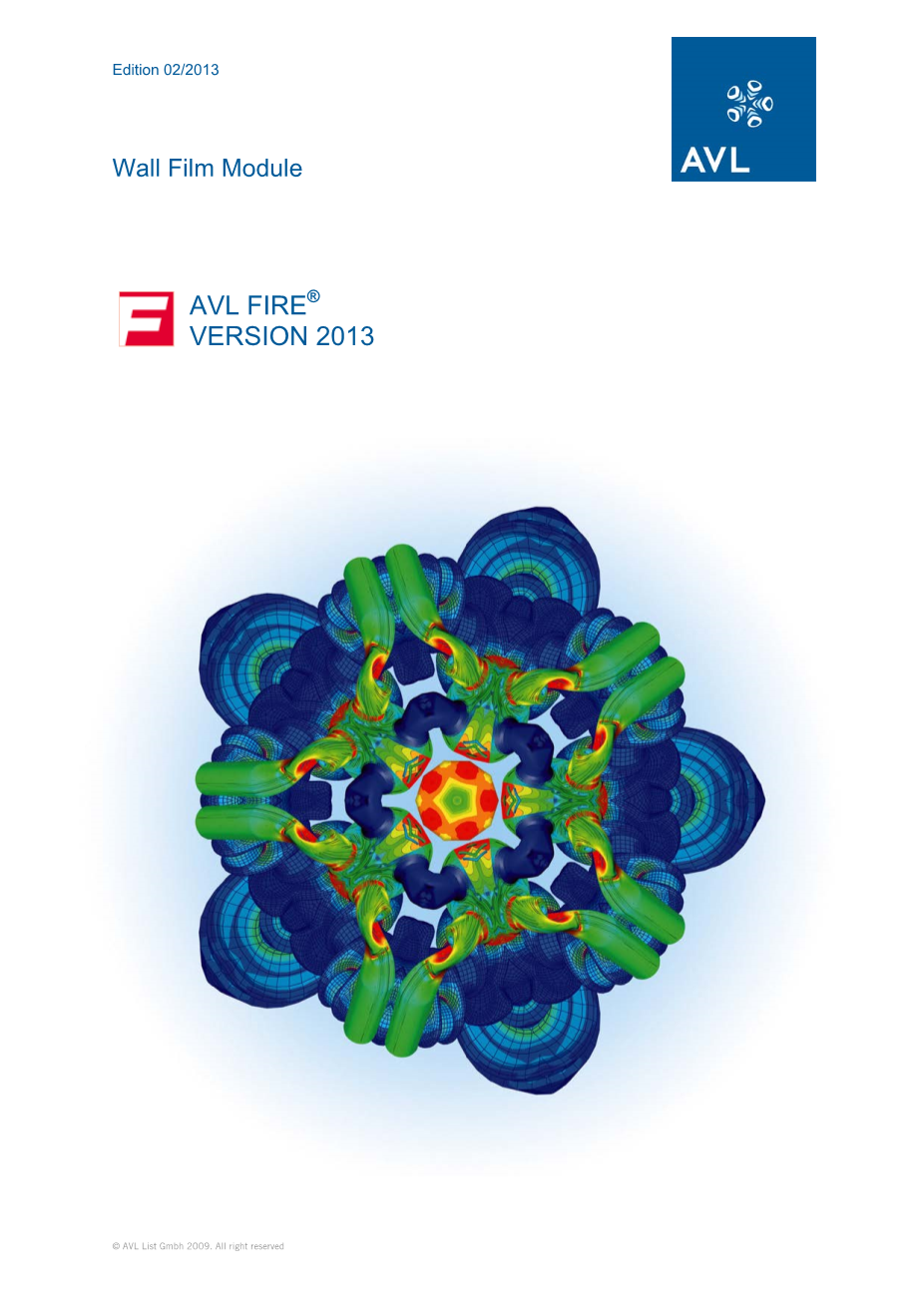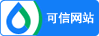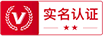### 注册

•• 获取手机验证码 60
• 注册

### 找回密码

•• 获取手机验证码60
• 找回

### 车用柴油机SCR系统排气管中沉积物的仿真研究外文翻译资料

2022-10-17 03:102.4.1.4. Multi-component Evaporation Model The multi-component evaporation is based on the Abramzon-Sirignano approach, which has been extended by Brenn et al. [2.13]. The main difference to the single-component case is that mass transfer of every component is taken into account separately, whereas heat transfer remains a global mechanism (Figure 2-2). The resulting mass flow rate then is the sum of the single contributions: （2.36） 2.4.1.4 多组分蒸发模型 本文使用多相蒸发模型对添蓝液滴的蒸发过程进行描述。该模型是在Abramzon-Sirignano 模型的基础上，分开考虑了每一组分的质量传递过程，而在描述热量传递过程时仍然将液滴看作整体，如图2-2 所示。 由此产生的质量流量是每一单一的组分的总和： （2.36） 其中，N指组分数量  

 The distribution of the components inside the droplet is assumed to be homogeneous. This assumption is justified for sufficiently high liquid mass diffusivities and moderate evaporation rates. That means, according to the “rapid mixing” approach the model is based on the assumption of a homogeneous liquid composition, which also reduces calculation time. From integration over the concentration boundary layer surrounding the droplet, one gets equations for the i-th component evaporation mass transfer rate. The modified Sherwood number is related to the standard Sherwood number Sh0 via the same correction function as given in the previous chapter for the single component case. It is evaluated with thermophysical properties for component i, i=1,...,N. （2.37） As given in the Spalding number for component i is （2.38） which is calculated with individual concentrations of component i at the drop surface and at infinity. Further in contrast to [2.13] the total droplet radius instead of the volume equivalent partial radius is used to assure consistency for the limiting case of same fuel in all components (see [2.28]). The mass transfer rate can also be calculated from an energy balance in the thermal boundary layer and subsequent integration. However, now thermophysical properties have been set up as average values over the components indicated below by an overbar. （3.39） The mass transfer rates are calculated using a similar iteration procedure as in the single component case. From the mass loss the new droplet mass, diameter, fuel composition as well as source terms for the fuel species transport equations are calculated. Finally using the equation given below the heat transferred to the drop is determined as （2.40） This enters the energy balance, from which the new drop temperature is calculated. The mole fractions of the components used to calculate mixture fractions and mixture properties at the drop surface are calculated using the activity coefficients . Thus real gas behavior is taken into account igamma; （2.41） instead of Raoultsrsquo;s law corresponding to=1.Considering a real liquid, the activity coefficient takes into account the interdependence of the various components due to shape and interaction forces between the molecules. From the various methods for the determination of gamma;i here the UNIFAC method is used, which is based on the molecular structure of the components [2.68 , 2.69]. More details about the implementation and validation of the model can be found in [2.28]. 2.4.2.7. Huh-Gosman Model This model assumes nozzle flow turbulence as decisive for the break-up mechanism and is based on the work of Huh and Gosman as well as Huh, Lee and Koo [2.40], [2.41]. The turbulent fluctuations are characterized by turbulent length and time scales. For the turbulent length and time scales Lt and tau;t algebraic formulae are used. （2.118） with C0 kept constant as C0=0.92. This value has been fitted for DGI multi-hole injection conditions. Initial values for the length and time scale C5 and C6 have to be provided as input parameters, which stand for initial turbulent length scale and turbulent time scale. Those can be calculated from average TKE and TED values in the nozzle cross section as shown above. Here KC indicates a form loss coefficient at the contraction corner with typical value of 0.45, constant Kt should be set to 0.27, s denotes the flow contraction coefficient with typical value of 0.9 and Cd is the nozzle discharge coefficient (0.7 to 0.9). In this model the break-up length scale LA is taken from the turbulent length scale Lt as （2.119） The break-up time scale tau;A is determined from a linear combination of the turbulent and aerodynamic time-scales （2.120） The aerodynamic time scale tau;W =f(LW ) is taken from a Kelvin-Helmholtz instability analysis using an aerodynamic length scale calculated from （2.121） The break-up rate is finally determined from the ratio of atomization length and time scales. （2.122） As in the WAVE or FIPA models child droplet production can be adjusted using two additional model parameters C7 and C8 in this model also. 2.4.7.5. Kuhnke/Wruck Wall Interaction and Wall Heat Transfer Models The model of Kuhnke [2.45] is an advanced wall interaction model, which in contrast to other models also takes into account the wall temperature TW besides the impact parameter K. It is used especially to describe the spray/wall interaction in SCR spray applications, but can also be applied elsewhere, when wall temperature is of importance. Depending on a dimensionless wall temperature （2.182） and a dimensionless droplet velocity （2.183） the Kuhnke model distinguishes between four regimes (see Figure 2-16): 液滴内部各组分的分布被认 剩余内容已隐藏，支付完成后下载完整资料 资料编号：，资料为PDF文档或Word文档，PDF文档可免费转换为Word

### 最新文档•